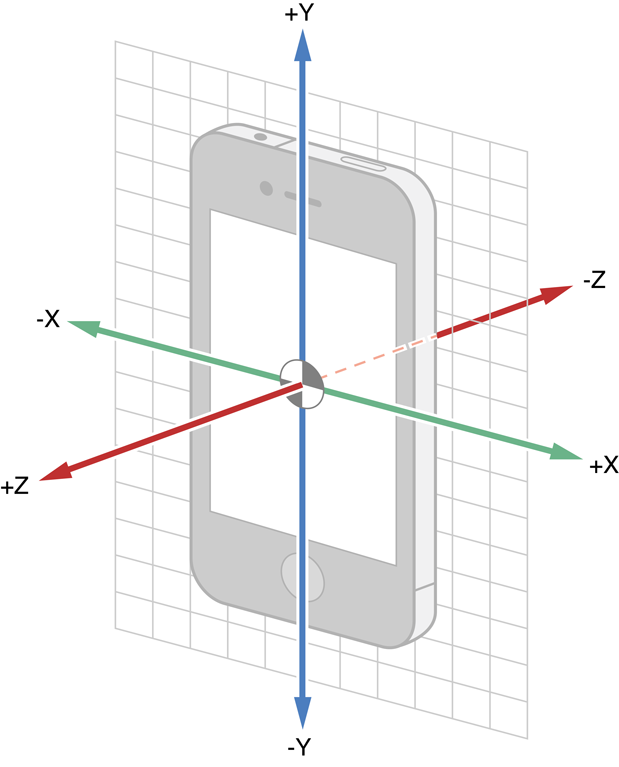Back to Blog Home← all posts

# Detecting Shakes in NativeScript

December 3, 2019 — by Alexander Vakrilov

In this blog we are going to learn how to detect a "shake" gesture in NativeScript apps using the nativescript-accelerometer plugin.## Getting Accelerometer Data

First of all we are going to use the nativescript-accelerometer plugin to start listening to accelerometer data:

import { startAccelerometerUpdates, AccelerometerData } from "nativescript-accelerometer";

console.log("x: " + data.x + "y: " + data.y + "z: " + data.z);
}, { sensorDelay: "ui" });


The AccelerometerData gives us the current accelerometer readings for each of the x, y, z axis. Because we passed sensorDelay: "ui" the plugin will give us reading approximately every 60ms, which seems to be enough for our purpose.Values we get are normalized. Value of 1 actually equals the Earth's acceleration (9.81 m/s2). This means if the phone is still, the AccelerometerData vector will point to the ground and its length ($\sqrt{x^2+y^2+z^2}$) will equal 1.

Note: This also means that if the phone is in a free-fall (or tossed in the air) the length of that vector will be zero as it will be in weightlessness. In summary: phone not moving -> vector length 1, phone falling -> vector length is zero. This is an interesting (and a little counter-intuitive) consequence of how accelerometers work.

## Detecting a Shake

Now, let's implement the shake detection.

Shaking the phone means that we are applying forces to it in different directions. We know from Newton's second law that $\vec{F}=m\vec{a}$ and this results in changes in the acceleration vector.

We are going to use the ShakeDetector implementation. It accepts a callback to be called when shake is detected and expect its onSensorData(data) to be called with values from the accelerometer. Starting/stopping accelemeter updates are left to the consumer of the class.

import { time } from "tns-core-modules/profiling";
import { AccelerometerData } from "nativescript-accelerometer";

const FORCE_THRESHOLD = 0.5;
const TIME_THRESHOLD = 100;
const SHAKE_TIMEOUT = 800;
const SHAKE_THROTTLE = 1000;
const SHAKE_COUNT = 3;

export class ShakeDetector {
private lastTime = 0;
private lastShake = 0;
private lastForce = 0;
private shakeCount = 0;
private cb: Function;

constructor(callback: () => void) {
this.cb = zonedCallback(callback);
}

public onSensorData(data: AccelerometerData) {
const now = time();
if ((now - this.lastForce) > SHAKE_TIMEOUT) {
this.shakeCount = 0;
}

const timeDelta = now - this.lastTime;
if (timeDelta > TIME_THRESHOLD) {
const forceVector = Math.abs(Math.sqrt(Math.pow(data.x, 2) + Math.pow(data.y, 2) + Math.pow(data.z, 2)) - 1);

if (forceVector > FORCE_THRESHOLD) {
this.shakeCount++;
if ((this.shakeCount >= SHAKE_COUNT) && (now - this.lastShake > SHAKE_THROTTLE)) {
this.lastShake = now;
this.shakeCount = 0;

this.cb();
}
this.lastForce = now;
}

this.lastTime = now;
}
}
}


The ShakeDetector will detect a shake if:

• register 3 readings (SHAKE_COUNT)
• that are separated by less than a 800ms (SHAKE_TIMEOUT)
• and that have the acceleration vector length differ from 1 (the constant acceleration we get from Earth's gravity) by at least 0.5 (FORCE_THRESHOLD)

If all these condition are met, we will call the callback and wait for 1 second (SHAKE_THROTTLE) before firing the event again to avoid firing the event multiple times during a long shake.

Interestingly enough, we don't even care about changes in the direction of the acceleration vector. This makes this algorithm a little inaccurate. If you remember the note from previous section - if the phone is falling, the acceleration vector will be 0 and our check will detected as a shake. However I found that in normal usage, it's hard to apply an acceleration of the phone in one direction consistently for a long time. You would have to change directions - which is actually a shake. So for practical usages it's good enough (unless you are in space 👨‍🚀).

## Putting it all Together

The only thing left to do is instantiate a ShakeDetector and notify it on accelermoeter updates:

const shakeDetector = new ShakeDetector(() => {

You can check the full demo in the nativescript-accelerometer repo.